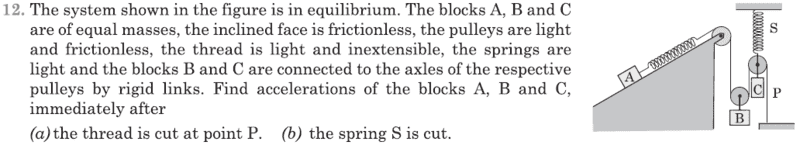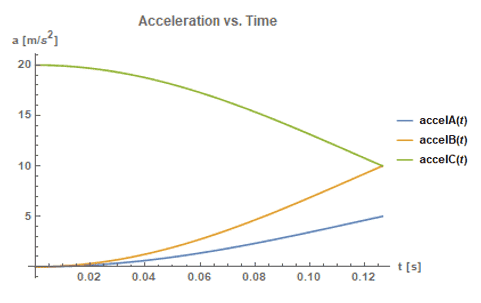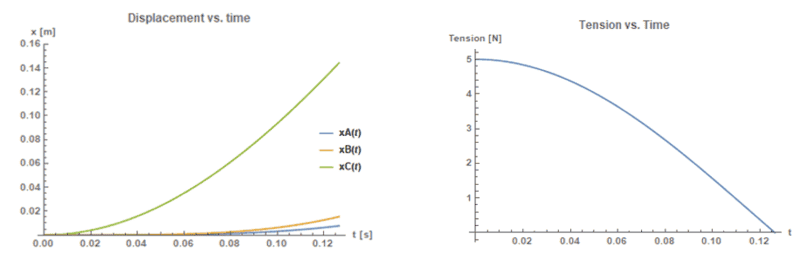# Tension in a complex-pulley-system

• Rikudo
In summary, the conversation discusses the tension around pulley C when spring S is cut. While the book claims that the tension remains and the acceleration of mass C is 2g, the speakers question this explanation and suggest that the system is not in equilibrium. They also discuss the possibility of the string going slack and the effects of the rigid connection between pulley C and mass C. Ultimately, they question the accuracy of the book's answers and the relationship between the spring and string tension.f

#### Rikudo

Homework Statement
See attachment.
Relevant Equations
-In equilibrium position, the pulley that holds mass C (we will call this pulley C later on) is pulled upwards by spring S . Hence, the string around the pulley C becomes tense. Now, if spring S is cut, my intuition says that the tension around this pulley will become zero, and mass C will undergo free fall. But, the book says the acceleration is 2g. I assume that it is because the tension is still there, but how is this possible?

Why do you think it would not be possible? It is the basic functioning mechanism behind a slingshot.

•nasu
Oh! So, we assume the string as the rubber in slingshot, but with a huge hooke's constant. When the whole system is at equilibrium , the string will be stretched infinitely small. After the spring is cut, it will return to its normal state, hence still exerts the tension. Am I correct?

So, we assume the string as the rubber in slingshot
No. The string is inextensible. The principle is the same due to the other spring.

I'm at a loss here. Could you please explain the relationship between that spring and the string tension?

•Delta2
The tension in the string is not going to go away just because you cut the other spring. You have to actually compute the tension. Generally the tension is going to result in the mass attached to the cut spring being accelerated more than g downwards.

If you draw a free body diagram of the system, with and without the force from S, you will see that forces are helping the pulley-mass C to fall faster than it would in free fall.

•Delta2
Hm. Does the tension on pulley C still there at time ##t## after the spring is cut?

Let me give a little explanation about why I think the tension is zero.

At the instant after the cut, The string will not in contact with pulley C.

It is the same as when we do the following activity:
1. We place to book on top of each other, and hold them at height,for example, x metres from the ground.
2. Release them

Each book will have acceleration g downwards because the contact force is not there anymore.

At the instant after the cut, The string will not in contact with pulley C.
I agree there is a flaw here. The problem is the connection between pulley C and mass C. It is shown as possibly a string. If so, cutting S will result in an arbitrarily large acceleration of pulley C and mass C going into free fall.
So take that connection to be a rigid rod. That makes the pulley and the mass effectively one object. Its inertia means the string over pulley C will not immediately go slack.

•Merlin3189 and Lnewqban
Now, if spring S is cut, my intuition says that the tension around this pulley will become zero, and mass C will undergo free fall. But, the book says the acceleration is 2g. I assume that it is because the tension is still there, but how is this possible?
Does the book give the answers for the accelerations of blocks A and B immediately after the spring is cut?

Does the book give the answers for the accelerations of blocks A and B immediately after the spring is cut?
Both are 0

Both are 0
Ok. At least that’s consistent with their answer for ##a_C##.

•Delta2
How could the system be in static equilibrium if the masses of blocks A and B are equal, and the slope is frictionless?

Hm. Does the tension on pulley C still there at time ##t## after the spring is cut?
Imagine an arrow being shot straight down after the hand releases it.
Would its vertical movement be a free fall?

•Merlin3189
Does the book give the answers for the accelerations of blocks A and B immediately after the spring is cut?

Both are 0
Ok. At least that’s consistent with their answer for ##a_C##.
I don't get it. Originally the masses are in equilibrium. This means that their velocities are zero before the spring is cut. Let's look at mass A on the incline. When the spring is cut, if the book's answer is correct and its acceleration is zero, the velocity of A will not change. If the velocity does not change from the zero, the position will not change and mass A will remain at rest on the frictionless incline. That's not happens when a mass is placed on a frictionless incline. I wouldn't trust the book's answers.

On edit: I got it. See posts 19-21.

Last edited:
Let's look at mass A on the incline. When the spring is cut, if the book's answer is correct and its acceleration is zero, the velocity of A will not change. If the velocity does not change from the zero, the position will not change and mass A will remain at rest on the frictionless incline. That's not happens when a mass is placed on a frictionless incline.
The acceleration and velocity of block A will be zero immediately after spring S is cut. But that doesn't mean that the acceleration of block A will remain zero.

Last edited:
The acceleration and velocity of block A will be zero immediately after spring S is cut. But that doesn't mean that the acceleration of block A will remain zero.
I disagree. As long as the spring is there, the acceleration is zero and the velocity is zero. Immediately after the spring is cut, the velocity is zero and the acceleration is ##g\sin\theta## down the incline. The free body diagram of mass A immediately after the spring is cut has two forces acting on mass A, gravity and the normal force. These two cannot add up to zero because they form an angle less than 180°. Therefore, the net force cannot be zero therefore the acceleration cannot be zero. My understanding of "cut" is that the spring no longer exerts a force on mass A and must therefore be removed from the FBD of mass A. What is yours?

On edit: All this is correct abotut the spring connected to mass A. That is not the spring that is cut.

Last edited:
I disagree. As long as the spring is there, the acceleration is zero and the velocity is zero. Immediately after the spring is cut, the velocity is zero and the acceleration is ##g\sin\theta## down the incline. The free body diagram of mass A immediately after the spring is cut has two forces acting on mass A, gravity and the normal force. These two cannot add up to zero because they form an angle less than 180°. Therefore, the net force cannot be zero therefore the acceleration cannot be zero. My understanding of "cut" is that the spring no longer exerts a force on mass A and must therefore be removed from the FBD of mass A. What is yours?
The spring being cut is the spring attached to the pulley in which C hangs, not the spring above the incline that attaches to A.

I don't get it. Originally the masses are in equilibrium. This means that their velocities are zero before the spring is cut. Let's look at mass A on the incline. When the spring is cut, if the book's answer is correct and its acceleration is zero, the velocity of A will not change. If the velocity does not change from the zero, the position will not change and mass A will remain at rest on the frictionless incline. That's not happens when a mass is placed on a frictionless incline. I wouldn't trust the book's answers.
You have to be careful not to consider too long a period. Clearly the blocks will move over time, but their instantaneous accelerations can be zero. It will become clearer if you analyse a period Δt then let that tend to zero.
The tension cannot change until the other spring shortens a bit.

•Orodruin
Its inertia means the string over pulley C will not immediately go slack.

1. We place to book on top of each other, and hold them at height,for example, x metres from the ground.
2. Release them

Each book will have acceleration g downwards because the contact force is not there anymore.

Wait, does that mean the inexistence of contact force between the books after we let them go is wrong?

Wait, does that mean the inexistence of contact force between the books after we let them go is wrong?
No, it means you are wrong to claim there is no contact force once the spring is cut, so it is not like your book example.
The instant after S is cut, the other spring is still at the same extension, so still exerts the same force, ½mg, on the string. That leads to total upward force from the string of mg on B, and a total downward force from the string of mg on pulley C. Assuming pulley C and mass C are rigidly connected, that gives a downward acceleration of 2g for C.

Regarding my example... Isn't inertia supposed to make the objects remain in the same place where we release them (which means, the contact force still exist)?

Regarding my example... Isn't inertia supposed to make the objects remain in the same place where we release them (which means, the contact force still exist)?
Inertia is the resistance to acceleration to an applied net force; it will not completely prevent acceleration.
Because the force of gravity in a gravitational field is proportional to the mass it acts on, that means the books will, in the absence of any other force, undergo the same acceleration.
Before release, the books have a normal force between them, leading to a small degree of compression. When released, that compression still exists at first, so the lower book accelerates down slightly faster, separating them. But then the lower one experiences more drag, so the books do stay together, but with a much smaller normal force.

•Lnewqban
When released, that compression still exists at first, so the lower book accelerates down slightly faster, separating them.

How come the same could not be applied to the string and pulley C in this problem?
Its inertia means the string over pulley C will not immediately go slack.

Edit: There might be an ambiguity in what kind of similarity between both of the cases. So, I'm going to elaborate a little.

After the cut, the tension will push pulley C downward a little. As a result, in duration of time ##dt##, the string will not become tout anymore.

Last edited:
The spring being cut is the spring attached to the pulley in which C hangs, not the spring above the incline that attaches to A.
Oops. Wrong spring. All is clear now. Sorry for butting in.

in duration of time dt, the string will not become tout anymore.
Exactly. Even if it does lose some tension, that requires the other spring to shorten. Since that involves accelerating masses, it is not instantaneous.
So, at first, the tension is almost unchanged, and the instantaneous acceleration of mass C is 2g.

Setting up and solving the equations of motion for the three masses is not too hard unless I’m overlooking something. Then we can look at how long it takes for the string to go slack after spring S is cut.

Positive direction for A is down the incline and positive directions for B and C are vertically downward. I find that if spring S is cut at time ##t = 0##, the accelerations of the blocks as functions of time turn out to be the following: $$a_A = \frac{4}{9} g \left[ 1-\cos(3 \omega t) \right]$$ $$a_B = 2a_A = \frac{8}{9} g \left[ 1-\cos(3 \omega t) \right]$$ $$a_C = 2g-a_B = \frac{1}{9} g \left[10 + 8 \cos(3 \omega t) \right]$$ Here, ##\omega \equiv \sqrt{k/m}##, where ##k## is the spring constant of the spring attached to A and ##m## is the mass of each block. Once the string goes slack these equations no longer apply.

The tension in the string becomes zero when ##a_B = a_C = g## and ##a_A = g/2##. This occurs at the time $$t_{slack} = \frac{\arccos(- 1/8)}{3 \omega} \approx 0.565\sqrt{\frac{m}{k}}$$
As an example, if I choose ##g = 10## m/s2, ##m = 1## kg and ##k = 20## N/m, then ##t_{slack} \approx 0.126## s. For this example, I get the following graphs for the accelerations of the blocks up until the string becomes slack:Here are graphs of the displacements of the blocks from their equilibrium positions and a graph of the tension in the string as functions of time:By the time the string becomes slack, block C has moved about 14.4 cm while blocks A and B have moved only about 1.5 cm and 0.8 cm, respectively.

Of course, all of this depends on whether or not my analysis is correct.

Last edited:
•Lnewqban, haruspex and Delta2